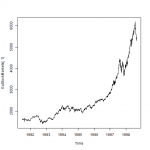Want to share your content on R-bloggers? click here if you have a blog, or here if you don't.Uncertainty is the biggest enemy of a profitable business. That is especially true of small business who don’t have enough resources to survive an unexpected diminution of revenue or to capitalize on a sudden increase of demand. In this context, it is especially important to be able to predict accurately the change in the markets to be able to make better decision and stay competitive.

This series of posts will teach you how to use data to make sound prediction. In the last set of exercises, we’ve seen how to make predictions on a random walk by isolating the white noise components via differentiation of the term of the time series. But this approach is valid only if the random components of the time series follow a normal distribution of constant mean and variance and if those components are added together in each iteration to create the new observations.

Today, we’ll see some transformations we can apply on the time series make them stationary, especially how to stabilise variance and how to detect and remove seasonality in a time series.

To be able to do theses exercise, you have to have installed the packages `forecast` and `tseries`.

Answers to the exercises are available here.

Exercise 1
Use the `data()` function to load the `EuStockMarkets` dataset from the R library. Then use the `diff()` function on `EuStockMarkets[,1]` to isolate the random component and plot them. This differentiation is the most used transformation with time series.

We can see that the mean of the random component of the time series seems to stay constant over time, but the variance seems to get bigger near 1997.
Exercise 2
Apply a the `log()` function on `EuStockMarkets[,1]` and repeat the step of exercise 1. The logarithmic transformation is often used to stabilise non constant variance.

Exercise 3
Use the `adf.test()` function from the `tseries` package to test if the time series you obtain in the last exercise is stationary. Use a lag of 1.

Exercise 4
Load and plot the `co2` dataset from the R library `dataset`. Use the `lowess()` function to create a trend line and add it to the plot of the time series.

By looking at the last plot, we can see that the time series oscillate from one side to the other of the trend line with a constant period. That characteristic is called seasonally and is often observed in time series. Just think about temperature, which change predictably from season to season.
Exercise 5
To eliminate the upward trend in the data use the `diff()` function and save the resulting time series in a variable called `diff.co2`. Plot the autocorrelation plot of `diff.co2`.

Exercise 6
This last autocorrelation plot has years for unit which is not really intuitive in our scenario. Make another autocorrelation plot where the x axis has months as units. By looking at this plot, can you tell what is the seasonal period of this time series?

Another way to verify if the time series show seasonnality is to use the `tbats` function from the forecast package. As his named says, this function fits a tbats model on the time series and return a smooth curve that fit the data. We’ll learn more about that model in a future post.
Exercise 7
Use the `tbats` function on the `co2` time series and store the result in a variable called `tbats.model`. If the time series show sign of seasonality, the `\$seasonal.periods` value of `tbats.model` will store the period value, else the result will be null.

Exercise 8
Use the `diff()` function with the appropriate lag to remove the seasonality of the `co2` time series, then use it again to remove the trend. Plot the resulting random component and the autocorrelation plot.

Exercise 9
Apply the adf test, the kpss test and the Ljung-Box test on the result of the last exercise to make sure that the random component is stationary.

Exercise 10
An interesting way to analyse a time series is to use the `decompose()` function which uses a moving average to estimate the seasonal, random and trend component of a time series. With that in mind, use this function and plot each component of the `co2` time series.